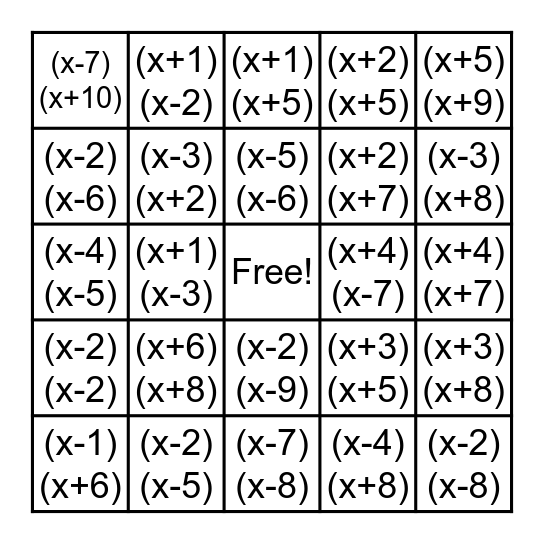# Factoring BingoThis bingo card has a free space and 39 words: (x+4)(x-8), (x-2)(x-10), (x-8)(x+10), (x-3)(x+2), (x-1)(x+6), (x-2)(x-9), (x-5)(x-6), (x+3)(x+10), (x+5)(x+9), (x-2)(x-2), (x+1)(x-3), (x+2)(x+5), (x-2)(x-8), (x+4)(x+7), (x+4)(x-7), (x+6)(x+8), (x-2)(x+3), (x-7)(x+10), (x-6)(x+8), (x+3)(x+5), (x-2)(x-6), (x-7)(x-8), (x+2)(x+7), (x-3)(x+8), (x+2)(x+4), (x+4)(x+5), (x-8)(x-9), (x+4)(x-10), (x+2)(x-4), (x+1)(x-2), (x-4)(x-4), (x-1)(x+2), (x+1)(x+5), (x-2)(x-5), (x-4)(x+8), (x-4)(x+7), (x+3)(x+8), (x-4)(x-5) and (x+6)(x+10).

## Play Online